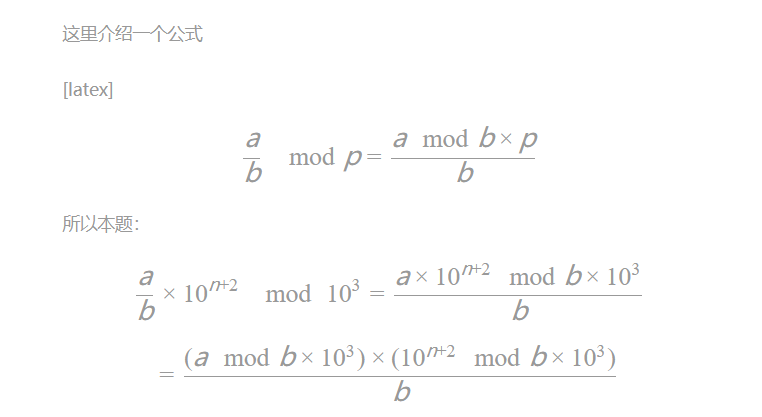# 蓝桥学习 PREV-52

** 试题 历届试题 **

PREV-52 小数第n位

如果我们把有限小数的末尾加上无限多个0，它们就有了统一的形式。

1 8 1

125

    一道看起来不是很难的题目，将a去除以b返回小数点第n个开始的三位数字，其实如果仅仅是这样难度也是不高的，只要先让a对b取余，之后每一次乘以10之后取余就行，但是由于本题的最后一个测试数据过于变态，用一般的方法必定会超时，所以得用一些特殊的方法。


    由知名弟弟[张伟杰](http://wp.tqtql8.top/)想到，如果每一次乘以10会超时那么将直接乘以10的10次方每一次让n-10，到小于10时再慢慢进行乘10取余


import java.util.Arrays;
import java.util.Scanner;
public class Main {
public static void main(String[] args) {
Scanner in=new Scanner(System.in);
long a,b,n;
a=in.nextLong();
b=in.nextLong();
n=in.nextLong();
while(n>10) {
a*=10000000000l;
a%=b;
n-=10;
}
while(n>1) {
a*=10;
a%=b;
n--;
}
a*=1000;
System.out.println(a/b);
}
}


    可以用判断目前的数是否与第一个数相同来判断小数的重复问题，从而达到剪枝的目的，虽然说可以通过后台但是算法本身是有问题的，而且判断第一个数来进行循环也是有转空子成分


#include<iostream>
using namespace std;
int ans(int a,int b)
{
cout<<a/b;
a%=b;
a*=10;
return a;
}
int main()
{
int a,b,n;
cin>>a>>b>>n;
int m=0;
a%=b;
int t = a;
while(n--)
{
if(a==b)break;
if(a<b)a=10;
else{
a%=b;
a=10;
if(a==0)break;
}
m++;
if(a%b==t)
{
n%=m;
}
}
if(a==0)cout<<"000"<<endl;
else{
for(int i=0;i<3;i++)
{
a=ans(a,b);
}
cout<<endl;
}
return 0;
}


    利用基础数论的知识，来计算逆元#include<iostream>
using namespace std;
typedef long long ll;
ll a,b,n,mod;
ll ksm(ll n,ll k)
{
ll ret=1;
while(k)
{
if(k&1) ret=((ret%mod)_(n%mod))%mod;
n=(n%mod)_(n%mod);
k>>=1;
}
return ret%mod;
}
int main()
{

cin>>a>>b>>n;
mod=b_1000;
ll son=(a%mod_ksm(10,n+2))%mod;
ll ans=son/b;
cout<<ans;
}

10-281708
01-3049007-3132
08-0126
08-0112
02-0845
04-29114
08-0113
08-0111
03-2372